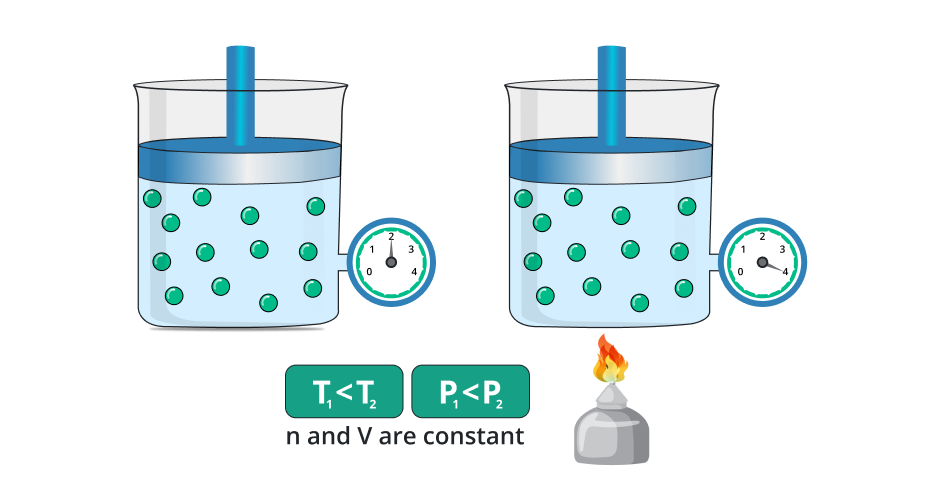# Gay Lussac's Law Calculator

To calculate the proportion of change in pressure (Pi, Pf) to change in temperature (Ti, Tf) enter values in the given boxes of Gay Lussac's Law Calculator and hit calculate button.

Formula:
PiPf = TiTf
kPa
K
kPa

Give Us Feedback

## Gay Lussac’s Law Calculator

Gay Lussac’s Law Calculator is a mathematical tool used to find pressure and temperature by using the ratio of pressure and temperature for initial and final pressure. It gives a step-by-step solution to every input problem.

## What is Gay Lussac’s Law?

This law tells us the change in the pressure of the gas due to the change in temperature by taking the quantity or amount of gas constant. In a simpler way if we change the temperature of the gas while keeping the amount of gas and volume constant then the pressure of the gas increases with respect to the temperature.

On the other hand, if we decrease the temperature of the gas then the pressure of the gas also decreases while taking the volume or amount of gas constant.In the general way, the law is stated as:

“It stated that the pressure of the gas is directly proportional to the temperature of the gas while taking the amount and volume of gas remain constant.”

### Formula:

The general formula of Gay Laussac’s law is written as.

`Pi/Pf  = Ti/Tf`

Where,

• Pi Initial Pressure
• PfFinal Pressure
• Ti = Initial temperature
• Tf = Final temperature

## How to use this Gay Lussac's Law calculator?

To calculate the proportion of pressure to the temperature follow the below steps.

1. Select the unknown value from the list let's suppose it is "Initial pressure (Pi)"
2. Input the remaining known values i-e Final pressure (Pf), Initial Temperature (Ti), and Final Temperature (Tf).
3. Press the "calculate" button.
4. Use the reset button to clear all the input fields.

After pressing the calculate button the unknown value of the initial pressure will be calculated according to Gay Lussac's Law.

## How to evaluate the pressure of the gas?

Here we solved the different examples to explain the tool and tell us how to find the temperature or pressure of the gas by using Gay Lussac’s Law.

Example 1:

Find the initial pressure of the gas if the initial temperature of the body is 23 kelvin after increasing the temperature up to 30 kelvin and the pressure of the gas changes to 50 K-Pascal.

Solution:

Step 1: Write the data from the question.

Pi = ? , initial temperature = Ti = 23 K, Final temperature = Tf = 30 K, Final Pressure = Pf = 50 K-Pa

Step 2: Write the formula of the Gay Lussac’s Law and arrange it according to the situation.

`Pi = Ti x Pf / Tf`

Step 3: By putting all values in the above formula and simplifying.

Ti = 23 K, Tf = 30 K, Pf = 50
Pi = Ti x Pf / Tf
Pi = 23 x 50 / 30
Pi = 1150 /30
Pi = 115 /3
`Pi = 38.33 k-Pa`

Example 2:

Evaluate the initial temperature of the gas if the initial pressure of the body is 23 K-Pascal after increasing the temperature up to 30 kelvin and the pressure of the gas changes to 50 K-Pascal.

Solution:

Step 1: Write the data from the question.

Ti = ? , initial pressure = Pi = 23 K, Final temperature = Tf = 30 K, Final Pressure = Pf = 50 K-Pa

Step 2: Write the formula of the Gay Lussac’s Law and arrange it according to the situation.

`Pi/Pf = Ti / Tf`

`Ti = Pi x Tf / Pf`

Step 3: By putting all values in the above formula and simplifying.

Pi = 23 K, Tf = 30 K, Pf = 50
Ti = Pi x Tf / Pf
Ti = 23 x 30 / 50
Ti = 690 /50
Ti = 69/5
`Ti = 13.8 k-Pa`

### Math Tools